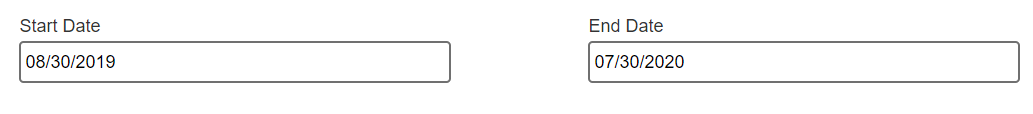1 comment

•I believe both the date fields you have in same form and the functionality should be once the first date is selected , the other date should be automatically get selected based on the calculation. (first date + 365 days )

We can achieve this using java script code . We can write a java script method which will do the calculation based on the selection of First date and input for how many days you want to add .

In the configuration window of Start Date on Change Event, you can call the above java script method . So this will trigger the method to calculate the new date every time you change the Start Date

So as per your requirement above , you want to add 365 days , which is like 1 year will get added to the selected date .

If you want to add few months or few dates , the calculation logic will change accordingly .

Here is a sample code snippet to achieve this .

```function addExtraDateToDependentDateField()
{
var startDate= \$('#StartDate').val();
var fUllDate= new Date(startDate);
var date= (fUllDate.getDate()) <= 9 ? "0" + (fUllDate.getDate()) : (fUllDate.getDate());
var month = (fUllDate.getMonth()) <= 9 ? "0" + (fUllDate.getMonth()) : (fUllDate.getMonth());
var year = fUllDate.getFullYear() + 1; // 365 days is 1 year
var endDate = month + "/" +date + "/" + year;
\$('#EndDate').val(endDate);
}Here is screenshot of the above code result.ThanksRoupya```